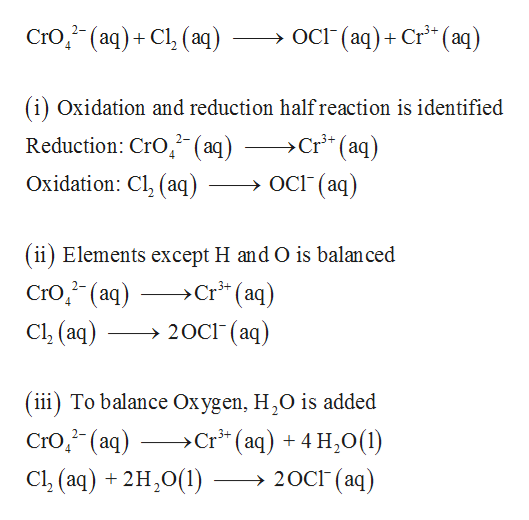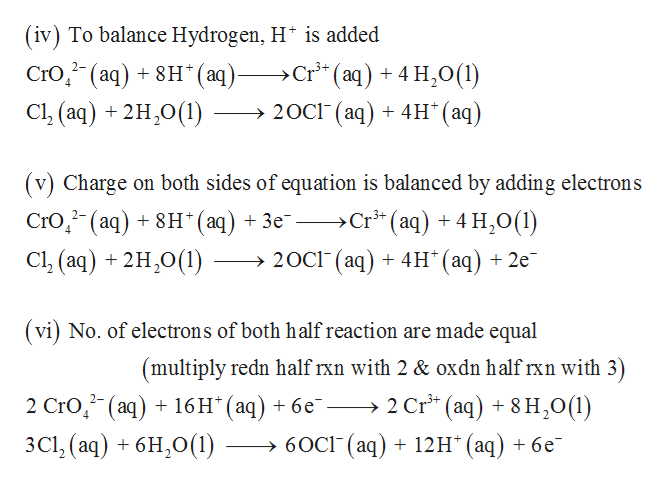# Balance the following redox reaction under acidic aqueous conditions using the smallest whole number coefficients possible CrO42-(aq)+Cl2(aq) ---> OCl-(aq)+Cr3+(aq)

Question
8 views

Balance the following redox reaction under acidic aqueous conditions using the smallest whole number coefficients possible

CrO42-(aq)+Cl2(aq) ---> OCl-(aq)+Cr3+(aq)

check_circle

Step 1

Seven steps are required to balance a redox reaction in acid solution. First reduction and oxidation half reaction are identified. The elements expect oxygen and hydrogen is balanced and then oxygen is balanced by adding H2O.help_outlineImage TranscriptioncloseOcl (aq)Cr (aq) CrO(aq)Cl (aq) - (i) Oxidation and reduction half reaction is identified >Cr* (aq) Reduction: CrO, (aq) 2- ОСt (aq) Oxidation: Cl, (aq) (ii) Elements except H and O is balan ced >Cr3 (aq) Cro(aq) 2- 20C1 (aq) Cl (aq) (iii) To balance Oxygen, H,O is added >Cr (aq) 4 H20(1) 20CI (aq) 3+ Стю," (аq) Cl (aq) +2H,O() fullscreen
Step 2

The hydrogen is balanced by adding H+ and then charge of each of the half reaction is balance...help_outlineImage Transcriptionclose(iv) To balance Hydrogen, H* is added >Cr3 (aq) 4 H,0(1) Cro, (aq) + 8н" (аq) — Cl, (aq) +2H,0() 2- 20C1 (aq)4H* (aq) Charge on both sides of equation is balanced by adding electrons Cro,- (aq) + 8н" (аq) + Зе —Cr* (aq) + 4 н,0(1) Cl (aq) +2H,0() 20CI (аq) + 4H" (ад) + 2е (vi) No. of electrons of both half reaction are made equal (multiply redn half rxn with 2 & oxdn half rxn with 3) 2 Cr (aq)8 H,0(1) 2 Cro, (aq) + 16H"(аq) + 6е - 3Cl (aq)6H20(1) >60CI (aq) + 12H* (aq) + 6e fullscreen

### Want to see the full answer?

See Solution

#### Want to see this answer and more?

Solutions are written by subject experts who are available 24/7. Questions are typically answered within 1 hour.*

See Solution
*Response times may vary by subject and question.
Tagged in

### Electrochemistry